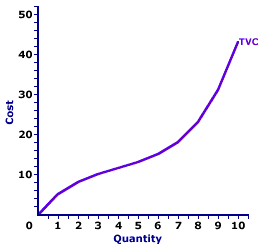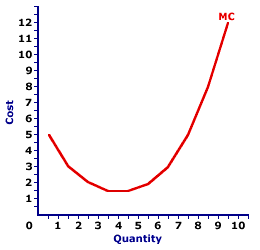Friday  December 8, 2023
 AmosWEB means Economics with a Touch of Whimsy!ZERO SLOPE: A horizontal line in which the numerical value of the slope, calculated as the change in the variable on the vertical axis divided by a change in the variable on the horizontal axis, is zero. In other words, the Y-axis variable is fixed, or constant, for any and all values of the X-axis variable.TOTAL VARIABLE COST AND MARGINAL COST:

A mathematical connection between marginal cost and total variable cost stating that marginal cost IS the slope of the total variable cost curve. This relation between total variable cost and marginal cost is also seen with total cost. The slope of the total cost curve is marginal cost, as well. The relation between total variable cost and marginal cost is but another in the long line of applications of the total-marginal relation.
Total Variable Cost and Marginal CostThe slope of the total variable cost curve (and total cost curve) is marginal cost. As such, if the total variable cost curve has a positive slope (that is, is upward sloping), then marginal cost is positive. Moreover, if the total variable cost curve has a positive and increasingly steeper slope, then the marginal cost is positive and rising. If the total variable cost curve has a positive and increasingly flatter slope, then the marginal cost is positive but falling.

This particular total-marginal relation applies to both total variable cost and total cost. Because not only is marginal cost the slope of the total variable cost curve, it is also the slope of the total cost curve. The reason is that any changes in total cost resulting from changing output is matched by changes in total variable cost. This occurs because total fixed cost is FIXED.

This two-paneled graph for the production of Wacky Willy Stuffed Amigos (those cute and cuddly armadillos, turtles, and lizards) visually illustrates the connection between total variable cost and marginal cost. For the first few quantities of output (Stuffed Amigos), total variable cost in the top panel is positive AND the slope of the total variable cost curve decreases--it becomes flatter. This corresponds with a positive and decreasing marginal cost in the bottom panel up to 4 Stuffed Amigos. For the next several quantities of Stuffed Amigos output, the slope of the total variable cost curve becomes increasingly steeper. This corresponds to an increasing marginal cost in the bottom panel.

The prime conclusion is the key role played by the law of diminishing marginal returns in the slope of both the marginal cost curve and the total variable cost curve.

• The U-shape of the marginal cost curve is a direct reflection of first increasing marginal returns, as marginal cost falls to a minimum, then decreasing marginal returns and the onset of the law of diminishing marginal returns as marginal cost rises.

• However, because the marginal cost curve is a plot of the slope of the total variable cost curve, then the shape of the total variable cost curve also reflects the law of diminishing marginal returns. The flattening slope of the total variable cost curve for small quantities of output is due to increasing marginal returns. Then the onset of the law of diminishing marginal returns causes the total variable cost curve to become increasingly steeper.
While this diagram relates marginal cost and total variable cost, the same story applies to the relation between marginal cost and total cost. Marginal cost is also the slope of the total cost curve. As such, the shape of the total cost curve is also a reflection of increasing, then decreasing marginal returns.

 <= TOTAL VARIABLE COST TOTAL VARIABLE COST AND TOTAL PRODUCT =>Recommended Citation:

TOTAL VARIABLE COST AND MARGINAL COST, AmosWEB Encyclonomic WEB*pedia, http://www.AmosWEB.com, AmosWEB LLC, 2000-2023. [Accessed: December 8, 2023].

Check Out These Related Terms...

Or For A Little Background...

And For Further Study...
Search Again?WHITE GULLIBON[What's This?] Today, you are likely to spend a great deal of time touring the new suburban shopping complex seeking to buy either a green and yellow striped sweater vest or a Boston Red Sox baseball cap. Be on the lookout for empty parking spaces that appear to be near the entrance to a store.Your Complete ScopeThe penny is the only coin minted by the U.S. government in which the "face" on the head looks to the right. All others face left."If you don't know where you are going, any road will get you there."-- Lewis Carroll, writerASAggregate SupplyA PEDestrian's Guide Xtra CreditTell us what you think about AmosWEB. Like what you see? Have suggestions for improvements? Let us know. Click the User Feedback link.| | | | | | | | | | |
| | | |

Thanks for visiting AmosWEB